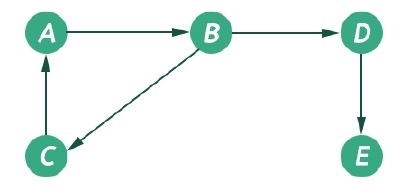# Graphs and its traversal algorithms

In this section we will see what is a graph data structure, and the traversal algorithms of it.

The graph is one non-linear data structure. That is consists of some nodes and their connected edges. The edges may be director or undirected. This graph can be represented as G(V, E). The following graph can be represented as G({A, B, C, D, E}, {(A, B), (B, D), (D, E), (B, C), (C, A)})The graph has two types of traversal algorithms. These are called the Breadth First Search and Depth First Search.

The Breadth First Search (BFS) traversal is an algorithm, which is used to visit all of the nodes of a given graph. In this traversal algorithm one node is selected and then all of the adjacent nodes are visited one by one. After completing all of the adjacent vertices, it moves further to check another vertices and checks its adjacent vertices again.

## Algorithm

bfs(vertices, start)
Input: The list of vertices, and the start vertex.
Output: Traverse all of the nodes, if the graph is connected.
Begin
define an empty queue que
at first mark all nodes status as unvisited
add the start vertex into the que
while que is not empty, do
delete item from que and set to u
display the vertex u
for all vertices 1 adjacent with u, do
if vertices[i] is unvisited, then
mark vertices[i] as temporarily visited
mark
done
mark u as completely visited
done
End

## Depth First Search (DFS)

The Depth First Search (DFS) is a graph traversal algorithm. In this algorithm one starting vertex is given, and when an adjacent vertex is found, it moves to that adjacent vertex first and try to traverse in the same manner.

## Algorithm

dfs(vertices, start)
Input: The list of all vertices, and the start node.
Output: Traverse all nodes in the graph.
Begin
initially make the state to unvisited for all nodes
push start into the stack
while stack is not empty, do
pop element from stack and set to u
display the node u
if u is not visited, then
mark u as visited
for all nodes i connected to u, do
if ith vertex is unvisited, then
push ith vertex into the stack
mark ith vertex as visited
done
done
End